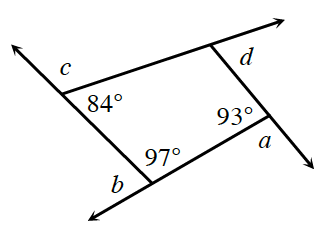### Home > CC1MN > Chapter 10 > Lesson 10.4.2 > Problem10-109

10-109.The exterior angles of a quadrilateral are labeled $a, b, c$, and $d$ in the diagram at right. Find the measures of $a, b, c,$ and $d$ and then find the sum of the exterior angles.

The missing exterior angles each form a straight angle with an interior angle.
What is the measure of a straight angle?

You will need to find the angle that forms a linear pair with $\angle d$.
If a quadrilateral can be made into two triangles, what is the sum of the interior angles of a quadrilateral?

$a = 87º, b = 83°, c = 96° d = 94º$,
$\angle a + \angle b + \angle c + \angle d = 360º$